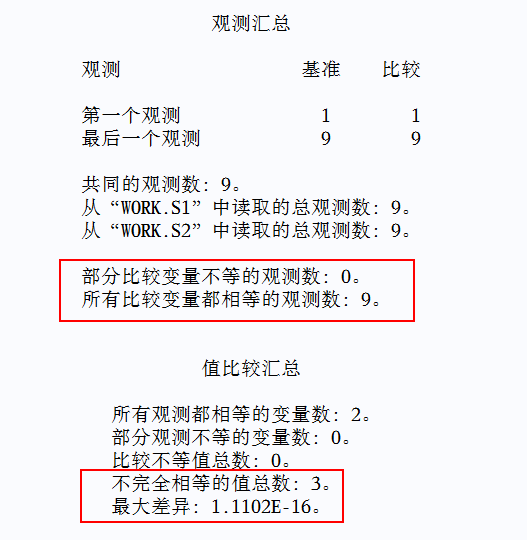# 1 数值存储方式

SAS使用8个字节存储数值，使用浮点计数法表示数值。

• 符号位：代表该数值是正数还是负数
• 基数：SAS系统中的基数默认为2。
• 指数：代表基数被乘的倍数
• 尾数：定义数值范围的一个小数# 2 产生的问题

（1）Truncation：2/3+2/3+2/3 = 0.666666 + 0.666666 + 0.666666 = 1.999998
（2）Rounding: 2/3+2/3+2/3 = 0.666667 + 0.666667 + 0.666667 = 2.000001

``````/*DO 迭代生成0.1~0.9*/
data s1;
do a = 0.1 to 0.9 by 0.1;
index + 1;
output;
end;
run;

/*手动输入0.1~0.9*/
data s2;
do b = 0.1, 0.2, 0.3, 0.4, 0.5, 0.6, 0.7, 0.8, 0.9;
index + 1;
output;
end;
run;

/*合并s1-s2*/
data s3;
merge s1 s2;
by index;
if a = b then A_eq_B = "Y";
run;

proc print data = s3;
var index a b A_eq_B;
run;
``````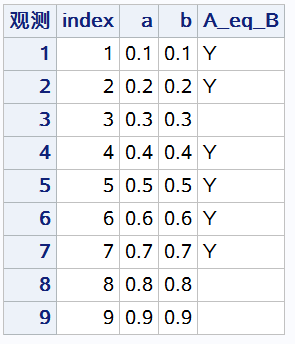``````proc print data = s3;
var index a b A_eq_B;
format a hex16. b hex16.;
run;
``````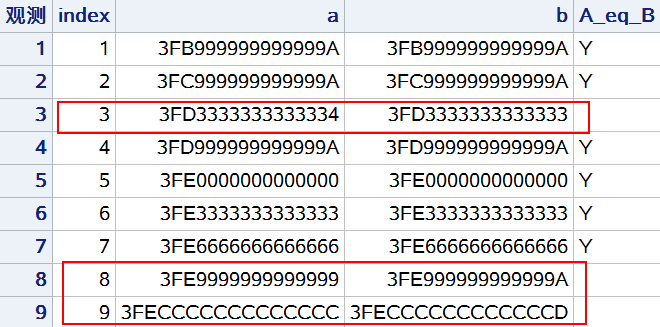• 使用 IF，SELECT，WHERE语句比较两个不同计算方法得出的数值，或与显式指定的数值比较时；
• 对数据集取子集时；
• 使用 PROC COMPARE 过程比较两个数据集时
• 对基于计算产生的浮点数进行分类分析时
• PROC REPORT或其他过程步的输出可能会显示出奇怪的小数（例如：-0.00）

# 3 解决办法

• 使用 ROUND 函数
• 为数值变量创建字符串版本的变量
• 利用过程步中的选项

（1）ROUND函数

``````data s3;
merge s1 s2;
by index;
a_r = round(a, 0.1);
b_r = round(b, 0.1);
if a_r = b_r then A_eq_B = "Y";
run;
proc print data = s3;
var index a b A_eq_B a_r b_r;
format a hex16. b hex16. a_r hex16. b_r hex16.;
run;
``````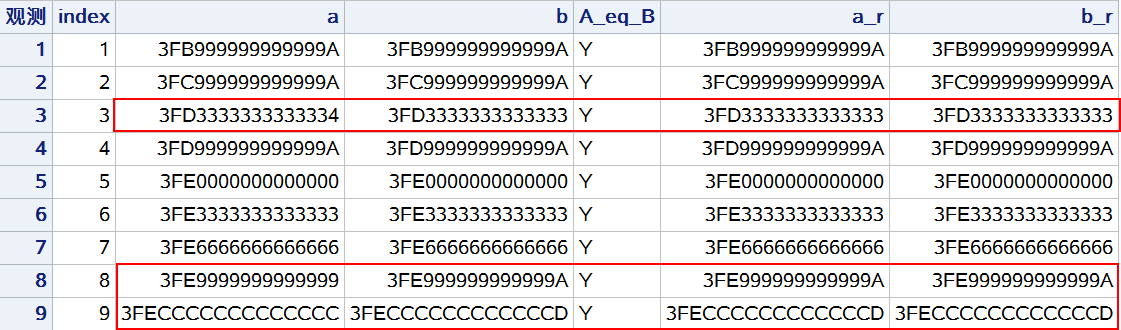（2）创建数值变量的字符串版本

``````data s3;
merge s1 s2;
by index;
a_fmt = put(a, 3.1);
b_fmt = put(b, 3.1);
if a_fmt = b_fmt then A_eq_B = "Y";
run;
proc print data = s3;
var index a b A_eq_B a_fmt b_fmt;
format a hex16. b hex16. a_fmt \$hex16. b_fmt \$hex16.;
run;
``````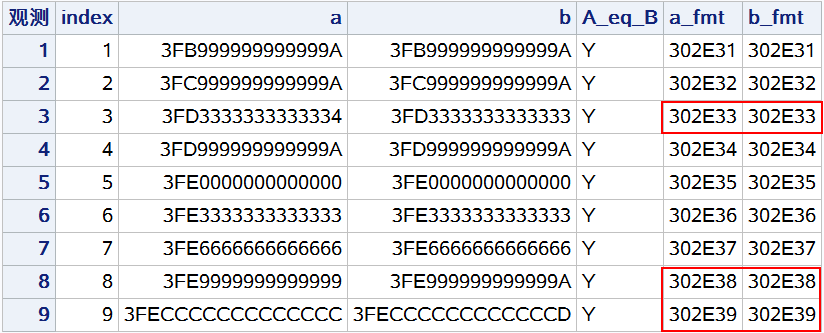（3）使用过程步中的选项

``````proc compare base = s1 compare = s2(rename = (b = a)) criterion = 0.1 method = absolute;
run;
``````